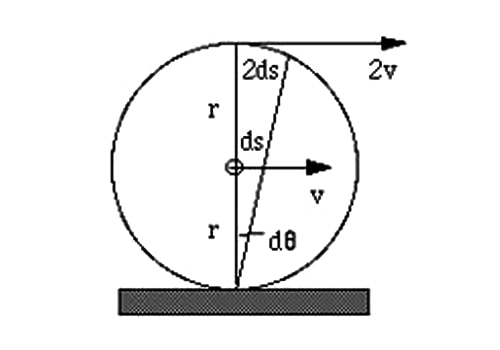# Condition for Skidding

When the centripetal force is greater than the frictional force, skidding occurs.

If μ is the coefficient of friction between the road and tire. then the limiting friction (frictional force) is f = μR where normal reaction R = mg

f = μ (mg)

Thus for skidding,

Centripetal force > Frictional forcemv2/r > μ (mg)

v2/rg > μ

but, v2/rg = tan θ

so, tan θ > μ

When the tangent of the angle of banking is greater than the coefficient of friction, skidding occurs.